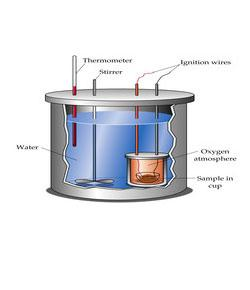# Mrs. Lawrence's Thermochemistry Quiz

Approved & Edited by ProProfs Editorial Team
The editorial team at ProProfs Quizzes consists of a select group of subject experts, trivia writers, and quiz masters who have authored over 10,000 quizzes taken by more than 100 million users. This team includes our in-house seasoned quiz moderators and subject matter experts. Our editorial experts, spread across the world, are rigorously trained using our comprehensive guidelines to ensure that you receive the highest quality quizzes.
| By MrsLawrence
M
MrsLawrence
Community Contributor
Quizzes Created: 2 | Total Attempts: 823
Questions: 5 | Attempts: 688SettingsThis quiz covers the terms endothermic, exothermic, Law of Conservation of Energy, heat of fusion, and heat of vaporization. It also contains a specific heat problem and a temperature conversion for degrees Celsius to Kelvin.

• 1.

### If a reaction releases heat (energy), it is referred to as

• A.

Endothermic

• B.

Exothermic

• C.

Isothermic

• D.

A chemical change

B. Exothermic
Explanation
If a reaction releases heat (energy), it is referred to as exothermic. This means that during the reaction, energy is being released from the system into the surroundings. The reaction is characterized by a decrease in the internal energy of the system, resulting in an increase in temperature of the surroundings. This energy release can often be observed as an increase in temperature, production of light, or formation of a flame.

Rate this question:

• 2.

### The heat absorbed by 50g of water to change from 10C to 30C is equal to

• A.

50 calories

• B.

500 calories

• C.

1000 calories

• D.

1000 joules

C. 1000 calories
Explanation
The heat absorbed by a substance can be calculated using the formula Q = mcΔT, where Q is the heat absorbed, m is the mass of the substance, c is the specific heat capacity of the substance, and ΔT is the change in temperature. In this case, the mass of water is given as 50g and the change in temperature is 30C - 10C = 20C. The specific heat capacity of water is approximately 1 calorie/g°C. Plugging in these values into the formula, we get Q = (50g)(1 calorie/g°C)(20C) = 1000 calories. Therefore, the correct answer is 1000 calories.

Rate this question:

• 3.

### The heat required to melt 1 gram of a solid at its melting point is called the

• A.

Specific heat capacity

• B.

Heat of vaporization

• C.

Law of Conservation of Energy

• D.

Heat of fusion

D. Heat of fusion
Explanation
The heat required to melt 1 gram of a solid at its melting point is called the heat of fusion. This term specifically refers to the amount of energy needed to change a substance from a solid to a liquid state, without changing its temperature. It is a measure of the strength of the bonds between the particles in the solid, as breaking these bonds requires energy. The heat of fusion is an important property to consider in processes such as melting or freezing substances.

Rate this question:

• 4.

### To convert degrees Celsius to degrees Kelvin, you

• A.

Multiply by 1.8 and add 32.

• B.

Subtract 32 and divide by 1.8.

• C.

• D.

Subtract 273.

Explanation
To convert degrees Celsius to degrees Kelvin, you need to add 273. This is because the Kelvin scale starts at absolute zero, which is -273 degrees Celsius. Adding 273 to the Celsius temperature will give you the equivalent temperature in Kelvin.

Rate this question:

• 5.

### Which of the following is NOT a unit for heat?

• A.

Celsius

• B.

Calories

• C.

Joules

• D.

Kilocalories

A. Celsius
Explanation
Celsius is a unit for temperature, not heat. Heat is measured in units such as calories, joules, and kilocalories. Celsius is used to measure the temperature of a substance or the amount of heat energy it contains, but it is not a unit for heat itself.

Rate this question:

Related TopicsBack to top